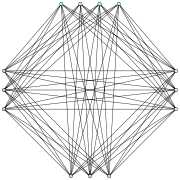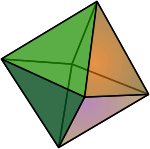Turán graph

﻿
Turán graph
Turán graphThe Turán graph T(13,4)
Named after Pál Turán
v · regular graph, if n is divisible by r.

Turán's theorem

Turán graphs are named after Pál Turán, who used them to prove Turán's theorem, an important result in extremal graph theory.

By the pigeonhole principle, any set of r+1 vertices in the Turán graph includes two vertices in the same partition subset; therefore, the Turán graph does not contain a clique of size r+1. According to Turán's theorem, the Turán graph has the maximum possible number of edges among all (r+1)-clique-free graphs with n vertices. Keevash and Sudakov (2003) show that the Turán graph is also the only (r+1)-clique-free graph of order n in which every subset of αn vertices spans at least$\frac{r\,{-}\,1}{2r}(2\alpha -1)n^2$ edges, if α is sufficiently close to 1. The Erdős–Stone theorem extends Turán's theorem by bounding the number of edges in a graph that does not have a fixed Turán graph as a subgraph. Via this theorem, similar bounds in extremal graph theory can be proven for any excluded subgraph, depending on the chromatic number of the subgraph.

Special casesThe octahedron, a cross polytope whose edges and vertices form a Turán graph T(6,3).

Several choices of the parameter r in a Turán graph lead to notable graphs that have been independently studied.

The Turán graph T(2n,n) can be formed by removing a perfect matching from a complete graph K2n. As Roberts (1969) showed, this graph has boxicity exactly n; it is sometimes known as the Roberts graph. This graph is also the 1-skeleton of an n-dimensional cross-polytope; for instance, the graph T(6,3) = K2,2,2 is the octahedral graph, the graph of the regular octahedron. If n couples go to a party, and each person shakes hands with every person except his or her partner, then this graph describes the set of handshakes that take place; for this reason it is also called the cocktail party graph.

The Turán graph T(n,2) is a complete bipartite graph and, when n is even, a Moore graph. When r is a divisor of n, the Turán graph is symmetric and strongly regular, although some authors consider Turán graphs to be a trivial case of strong regularity and therefore exclude them from the definition of a strongly regular graph.

The Turán graph$T(n,\lceil n/3\rceil)$ has 3a2b maximal cliques, where 3a+2b=n and b≤2; each maximal clique is formed by choosing one vertex from each partition subset. This is the largest number of maximal cliques possible among all n-vertex graphs regardless of the number of edges in the graph (Moon and Moser 1965); these graphs are sometimes called Moon-Moser graphs.

Other properties

Every Turán graph is a cograph; that is, it can be formed from individual vertices by a sequence of disjoint union and complement operations. Specifically, such a sequence can begin by forming each of the independent sets of the Turán graph as a disjoint union of isolated vertices. Then, the overall graph is the complement of the disjoint union of the complements of these independent sets.

Chao and Novacky (1982) show that the Turán graphs are chromatically unique: no other graphs have the same chromatic polynomials. Nikiforov (2005) uses Turán graphs to supply a lower bound for the sum of the kth eigenvalues of a graph and its complement.

Falls, Powell, and Snoeyink develop an efficient algorithm for finding clusters of orthologous groups of genes in genome data, by representing the data as a graph and searching for large Turán subgraphs.

Turán graphs also have some interesting properties related to geometric graph theory. Pór and Wood (2005) give a lower bound of Ω((rn)3/4) on the volume of any three-dimensional grid embedding of the Turán graph. Witsenhausen (1974) conjectures that the maximum sum of squared distances, among n points with unit diameter in Rd, is attained for a configuration formed by embedding a Turán graph onto the vertices of a regular simplex.

An n-vertex graph G is a subgraph of a Turán graph T(n,r) if and only if G admits an equitable coloring with r colors. The partition of the Turán graph into independent sets corresponds to the partition of G into color classes. In particular, the Turán graph is the unique maximal n-vertex graph with an r-color equitable coloring.